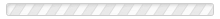Search:
(clear)
• c
• conjugate pairs
Conjugate pairs are pairs of properties to which the Heisenberg Uncertainty Principle applies. Conjugate pairs are also(...) Read More
• conservation laws
Physics includes several conservation laws. The most well-known is the Law of Conservation of Energy. This law states that(...) Read More
• conservation of energy
The Law of Conservation of Energy says that in our universe the amount of energy remains constant. Energy cannot be created(...) Read More
• conservation of matter
The Law of Conservation of Matter was abandoned as inaccurate by physicists early in the 1900’s. For more information, see(...) Read More
• conserved quantity
A conserved quantity is something that remains constant in amount over time and cannot be created nor destroyed. Conserved(...) Read More
• constant
A constant is a number or symbol that always has the same value. There are four types of constants: A mathematical(...) Read More
• constant of nature
A constant of nature is another way of saying a "physical constant." Read More
• Copenhagen Interpretation
The Copenhagen Interpretation of quantum mechanics is the original attempt by physicists to provide an explanation for the(...) Read More
• Coulomb's Law
Coulomb’s Law is a formula that allows us to calculate the electric force generated between two electrical charges.(...) Read More
• d
• dark energy
Dark energy is the name given to an unexplained aspect of the universe. Dark energy has been postulated as an explanation(...) Read More
• dark matter
"Dark matter" is a name that’s been given to a substance which many scientists believe may exist. If it exists, this(...) Read More
• de Broglie-Bohm interpretation of quantum mechanics
This interpretation of quantum mechanics is also called the "Bohmian Interpretation." It was originally proposed by(...) Read More
• decoherence
(Also called "quantum decoherence.”) Decoherence occurs when a quantum particle loses(...) Read More
• dimensionless number
Pi is an example of a dimensionless number. A dimensionless number doesn’t imply any measurement units. Pi is just 3.14, not(...) Read More
• dynamic system
A dynamic system is a system that changes over time. It can also be called a "dynamical system." While some maintain that(...) Read More
• dynamical system
• e
• e
e is the symbol for Euler's number. Similarly to pi, e is a constant which occurs repeatedly throughout nature. It has a(...) Read More
• Euler's number (e)
Euler's number, similarly to pi, is a constant which occurs repeatedly throughout nature. It is symbolized as e. It has a(...) Read More
• experimental physics
For experimental physics, see  theoretical physics. Read More
• f
• fine-structure constant
See alpha, , α.  Read More
• Free Will Theorem
Do quantum particles have free will? Can they make choices? Some physicists have considered this question seriously. But(...) Read More
• frequency
Frequency is the number of repeated motions in a period of time. These motions can be up and down, like a hammer, or back(...) Read More
• frequency and wavelength
Frequency and wavelength are both properties of waves. They are closely related, so this article discusses the two(...) Read More
• function
The term “function” is important in the field of quantum mechanics because is it the basis of the key term “wave function.”(...) Read More
• g
• Guiding Wave Interpretation of quantum mechanics
The Guiding Wave Interpretation of quantum mechanics is also called the "Bohmian Interpretation" as David Bohm was one of(...) Read More
• h
• Heisenberg Uncertainty Principle (HUP)
The Heisenberg Uncertainty Principle (HUP) is also called the “Indeterminacy Principle” or simply, the "Uncertainty(...) Read More
• HUP
“HUP” stands for the Heisenberg Uncertainty Principle, which see. Read More
• i
• i
The letter i is the symbol for the square root of -1. In other words i = √-1. The symbol i often appears in the equations of(...) Read More
• idealism
In ordinary English, "idealism" is contrasted with pragmatism. "Idealism" refers to the prioritizing of ideals. The meaning(...) Read More